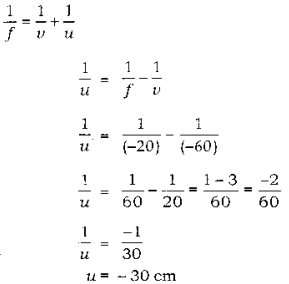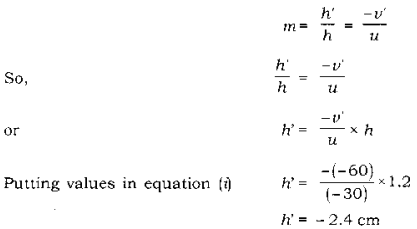# An object of height 1.2 m is placed before a concave mirror of focal length 20 cm

An object of height 1.2m is placed before a concave mirror of focal length 20cm so that a real image is formed at a distance of 60cm from it. Find the position of an object. What will be the height of the image formed?

Height of object, h = 1.2 cm

focal length of concave mirror, ‘f’ = - 20 cm
image distance, v = -60 cm
object distance, u = ?
height of image h’ = ?
Applying formulaAswe know that magnification (m) is equal toSo the height of the image is -2.4 cm.